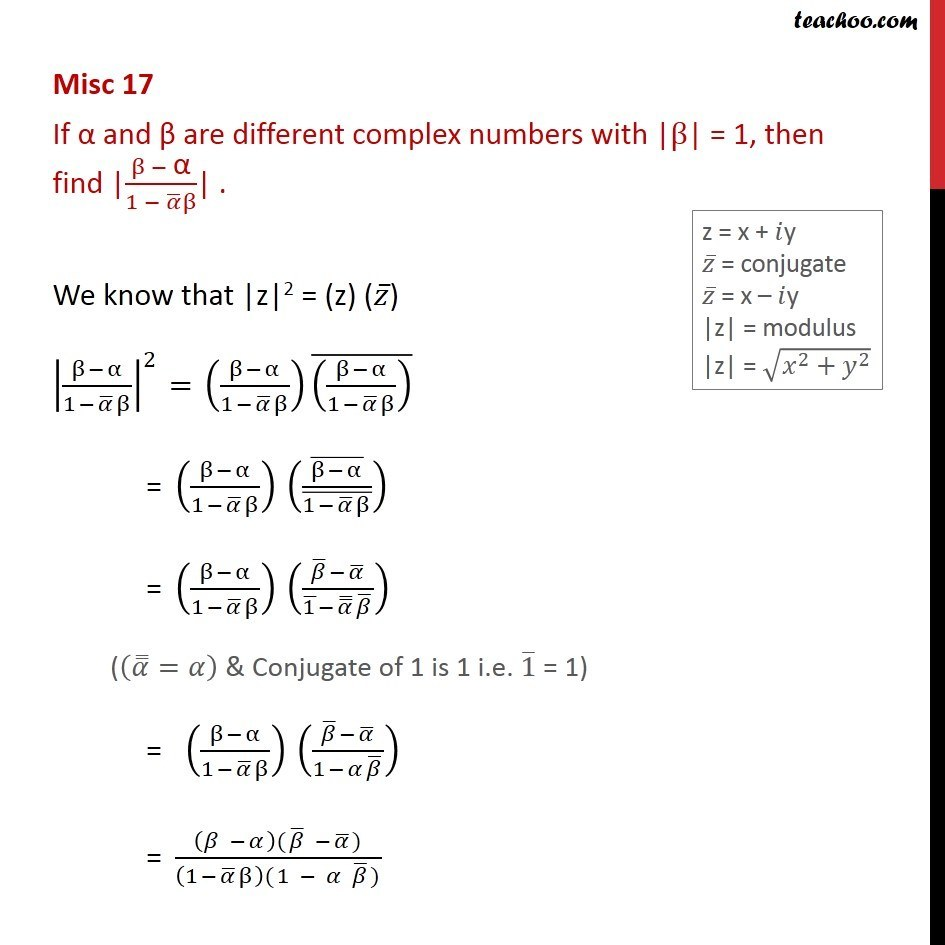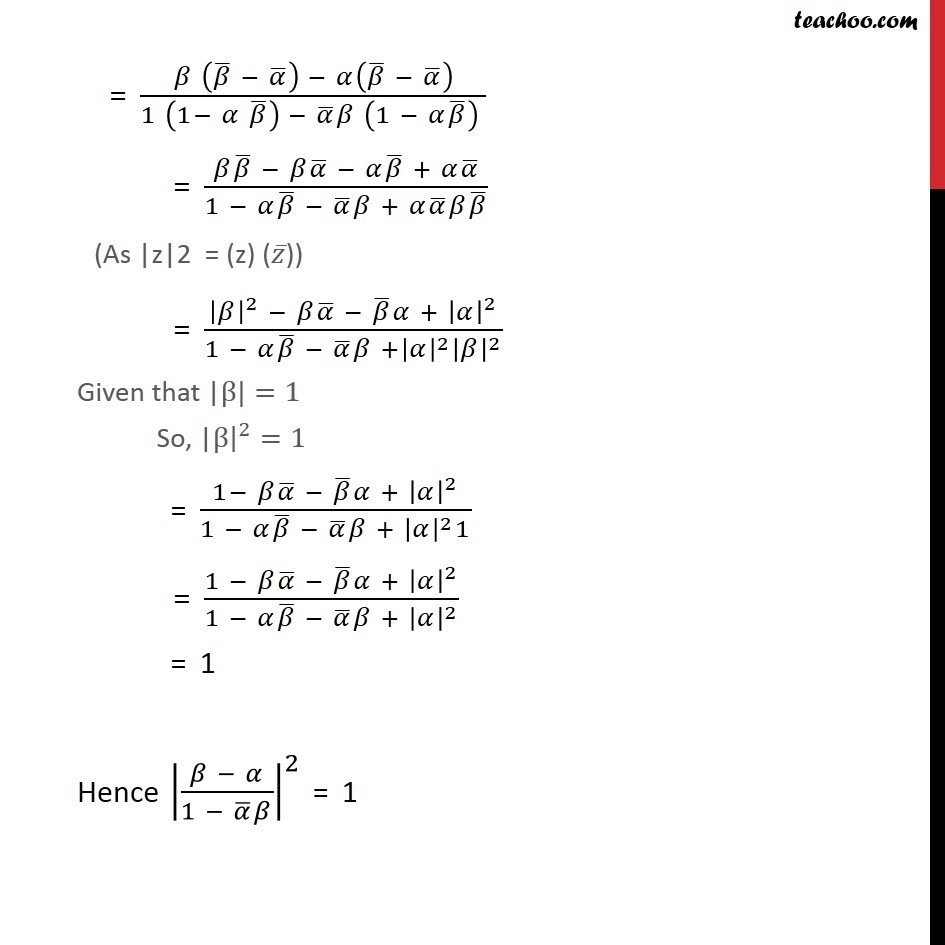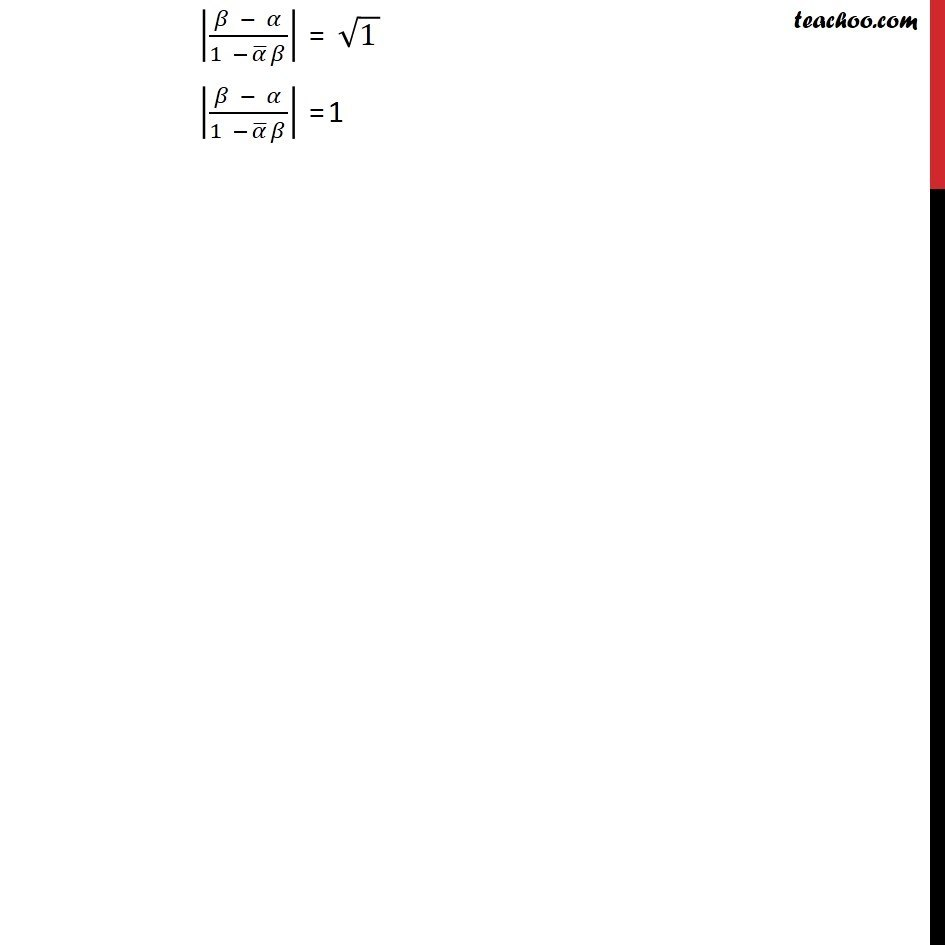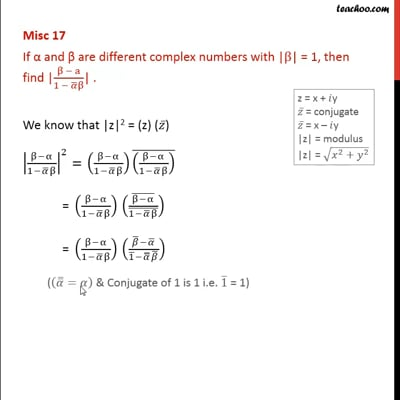Miscellaneous

Chapter 5 Class 11 Complex Numbers
Serial order wiseThis video is only available for Teachoo black users

Get live Maths 1-on-1 Classs - Class 6 to 12

### Transcript

Misc 17 If and are different complex numbers with | | = 1, then find |( " " )/(1 )| . We know that |z|2 = (z) ( ) |( )/(1 )|^2=(( )/(1 )) ((( )/(1 )) ) = (( )/(1 )) (( ) /(1 ) ) = (( )/(1 )) (( )/(1 )) = (( )/(1 )) (( )/(1 )) = (( )( ))/((1 )(1 )) = ( ( ) ( ))/(1 (1 ) (1 ) ) = ( + )/(1 + ) = (| |^2 + | |^2)/(1 +| |^2 | |^2 ) Given that | |=1 So, | |^2=1 = (1 + | |^2)/(1 + | |^2 1) = (1 + | |^2)/(1 + | |^2 ) = 1 Hence |( )/(1 )|^2 = 1 |( )/(1 )| = 1 |( )/(1 )| =1